# KSEEB Solutions for Class 5 Maths Chapter 9 Three Dimensional Figures

Students can Download Maths Chapter 9 Three Dimensional Figures Questions and Answers, Summary, Notes Pdf, KSEEB Solutions for Class 5 Maths helps you to revise the complete Karnataka State Board Syllabus and score more marks in your examinations.

## Karnataka State Syllabus Class 5 Maths Chapter 9 Three Dimensional Figures

### KSEEB Class 5 Maths Three Dimensional Figures Ex 9.1

Question a.
What is the shape of the faces of a cube?
Square

Question b.
How many circular faces are there in a cylinder?
TwoQuestion c.
Mention the number of plane surfaces and curved surfaces in a cone.
One, OneII. Observe the following table. Against each shape of solid, some pictures are given. Put (✓) against the figure which is an example of that shape and put (✗) against the figure which is not an example of that shape.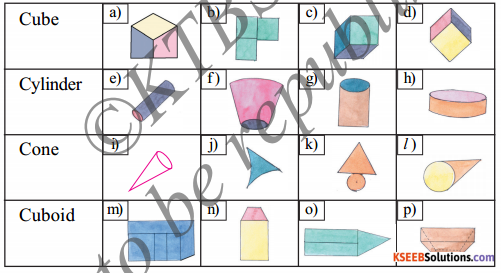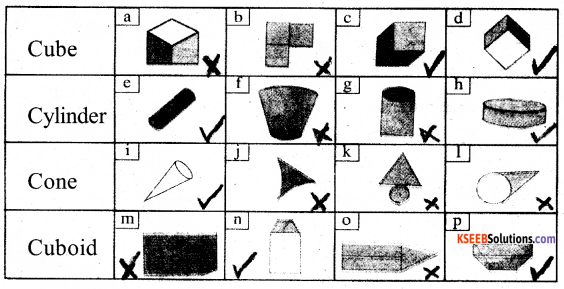III. A robot is constructed using different solid shapes. Identify the shapes and write the number of times it is used.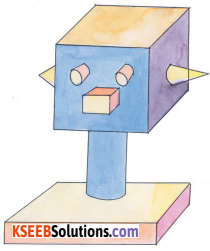Cube – 2, Cuboid -1, Cylinder – 3, Cone – 2IV. Different views of a table are given below. Name them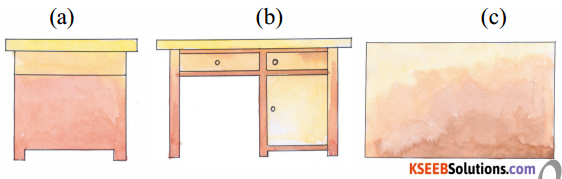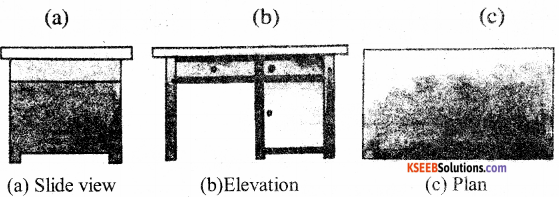V. 1. Draw diagrams of cube, cylinder and cone with different measurements in 2D.